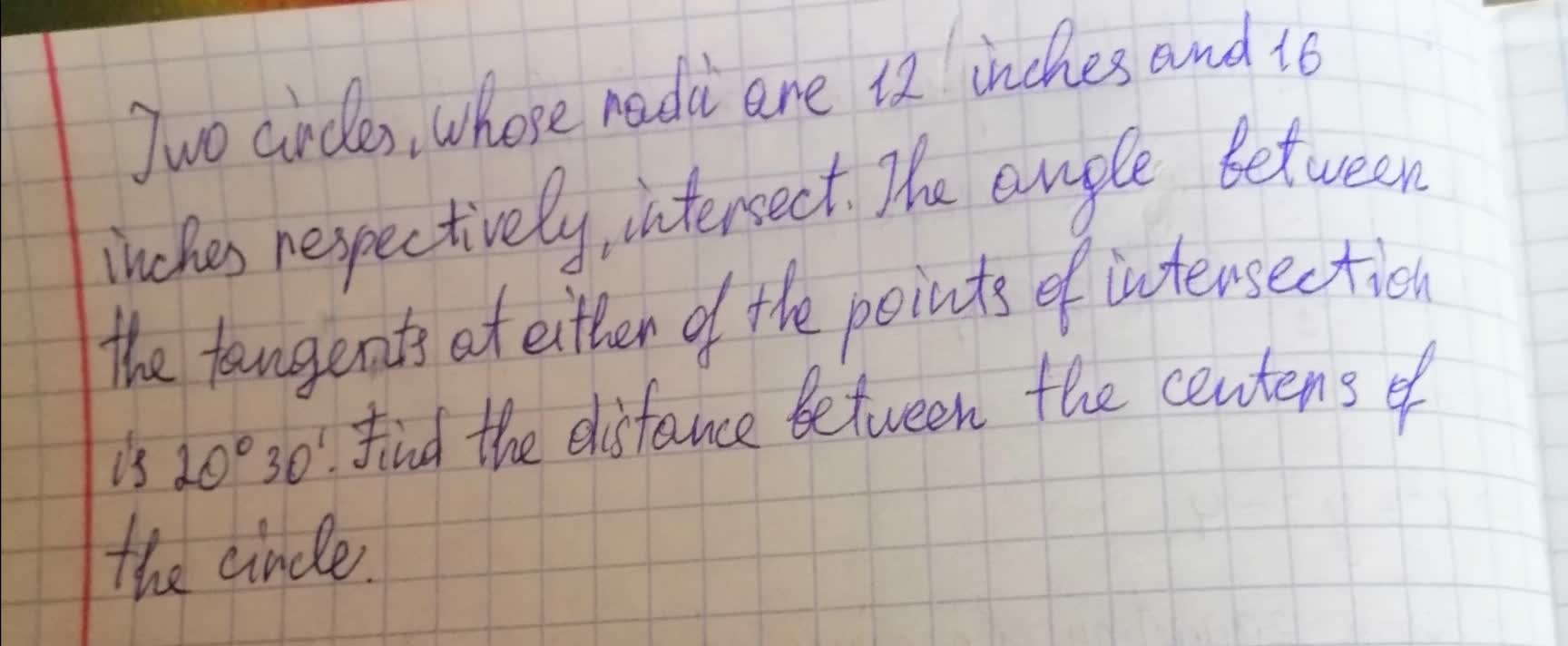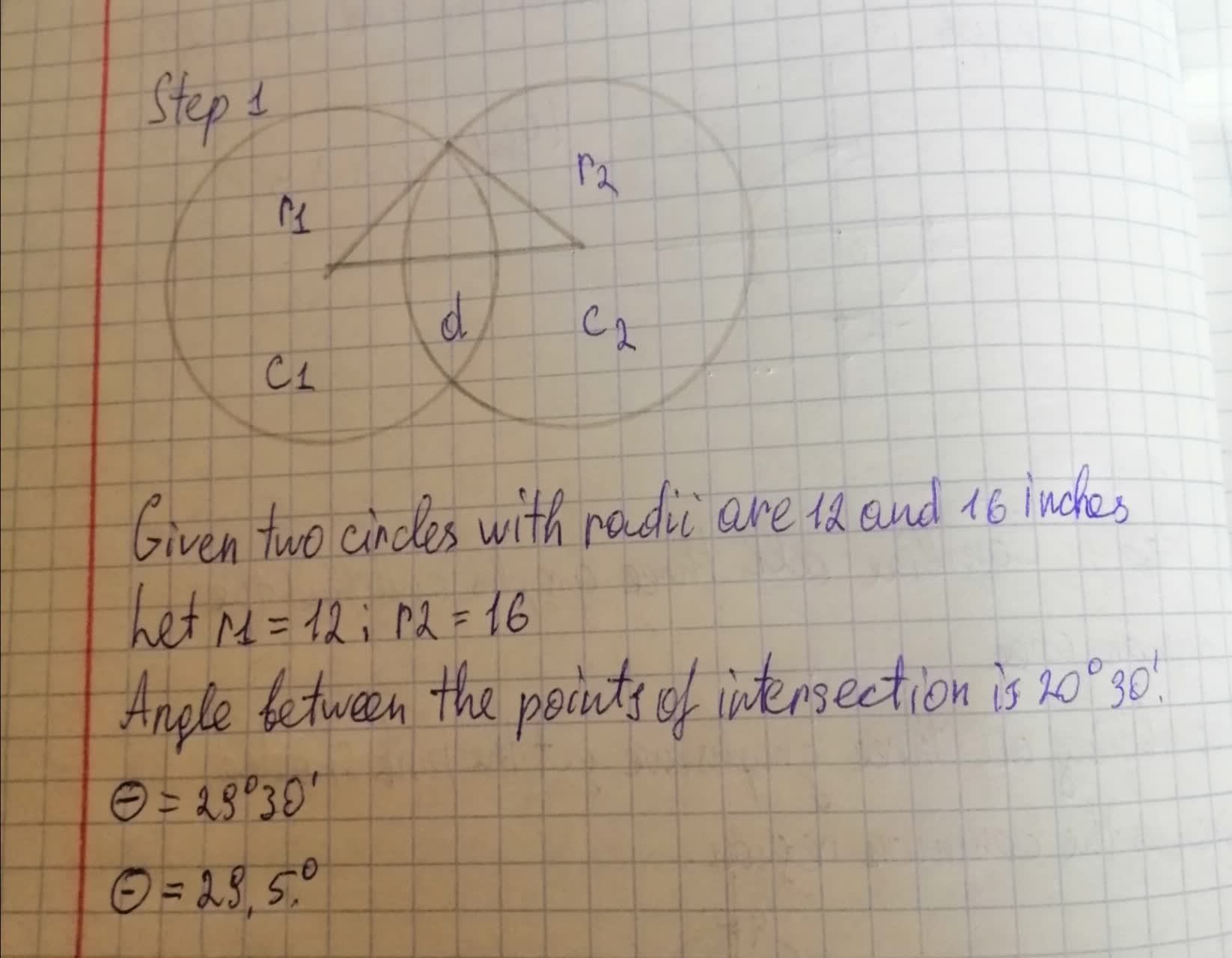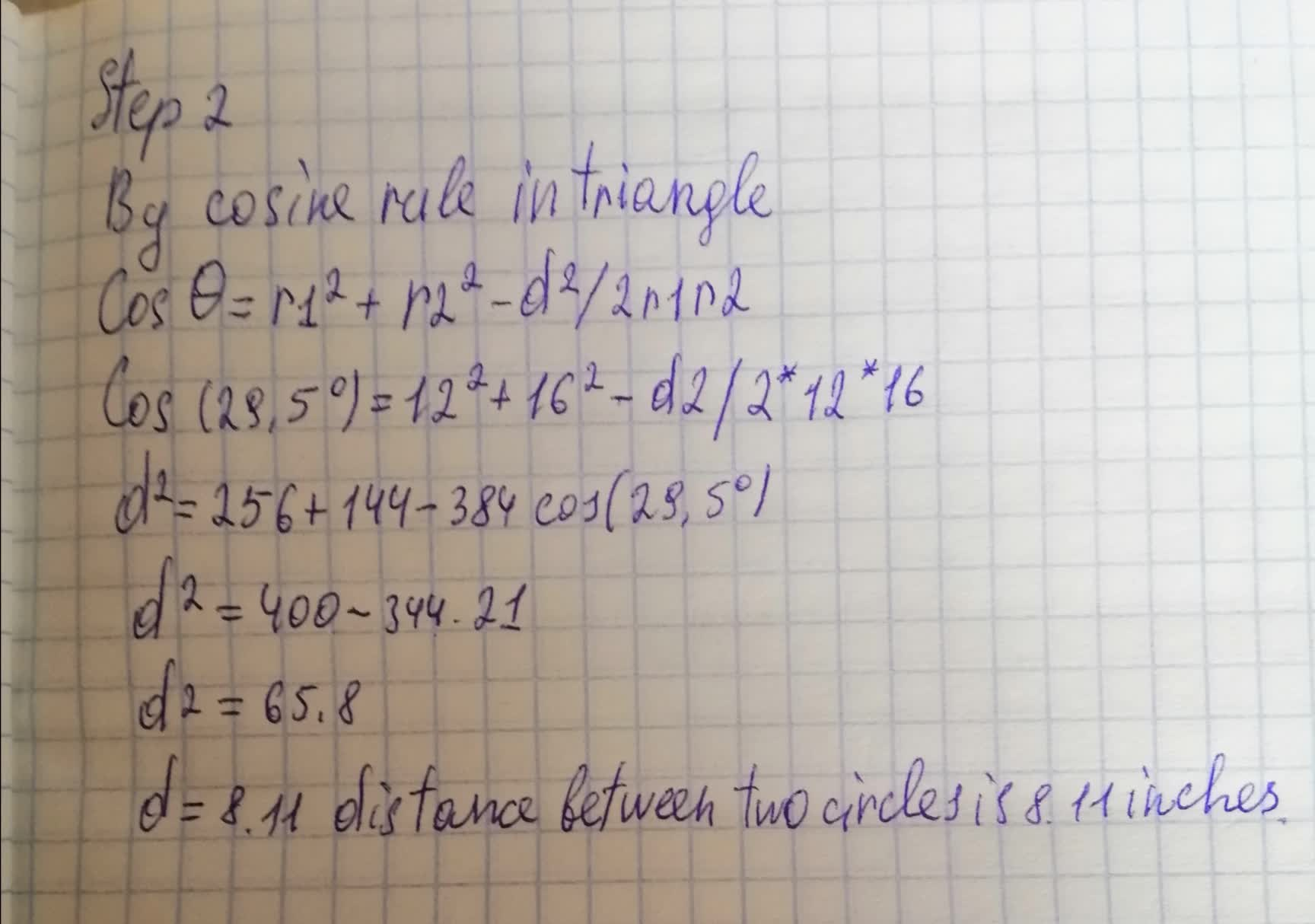# Two circles, whose radii are 12 inches and 16 inches respectively, intersect. The angle between the tangents at either of the points of intersection is 20^{\circ},\ 30'. Find the distance between the centers of the circle.snowlovelydayM 2021-08-14 Answered
Two circles, whose radii are 12 inches and 16 inches respectively, intersect. The angle between the tangents at either of the points of intersection is . Find the distance between the centers of the circle.You can still ask an expert for help

• Questions are typically answered in as fast as 30 minutes

Solve your problem for the price of one coffee

• Math expert for every subject
• Pay only if we can solve itwornoutwomanC

Consider in detail the answer to the photo